# Secondary Lecture

21 de Oct de 2015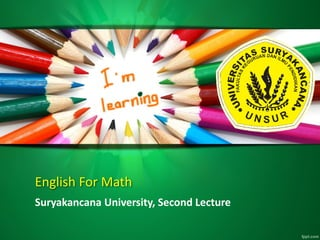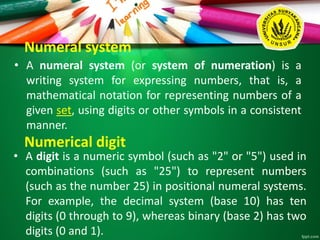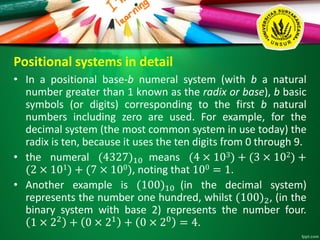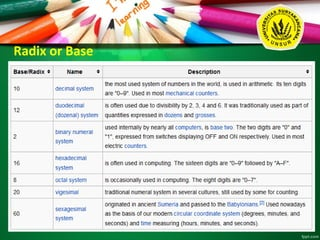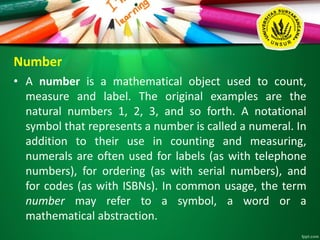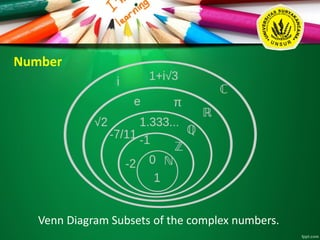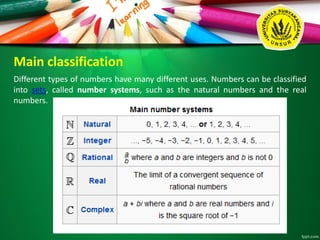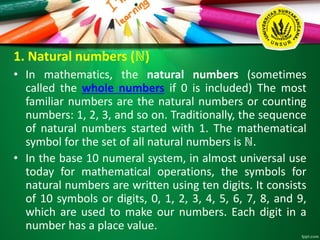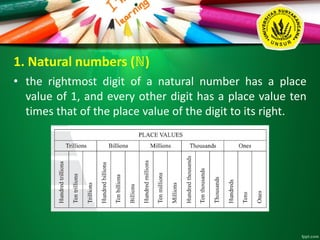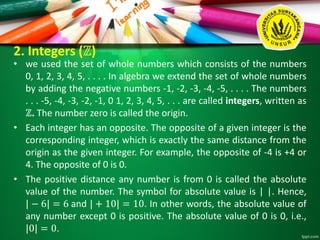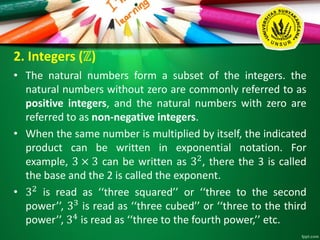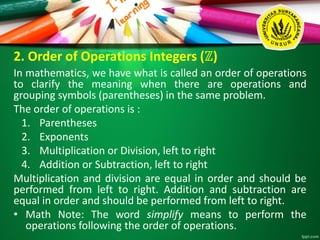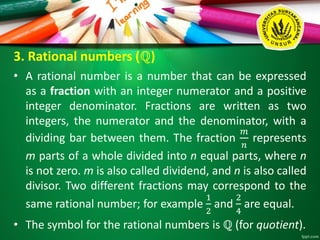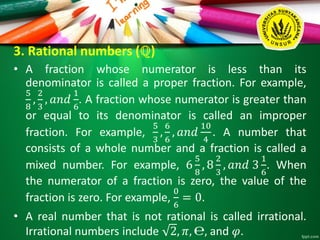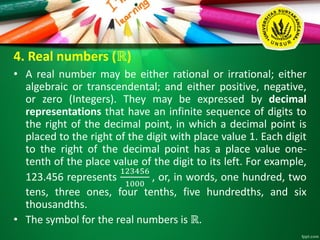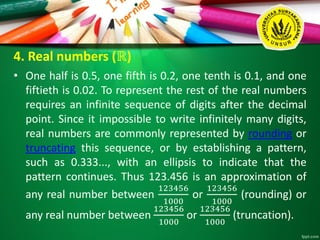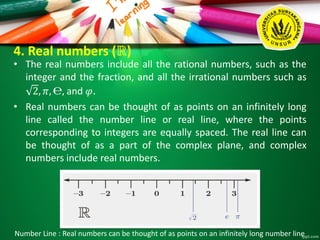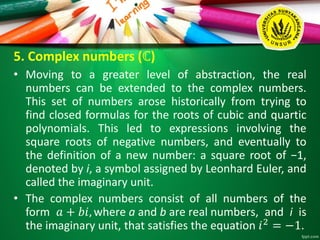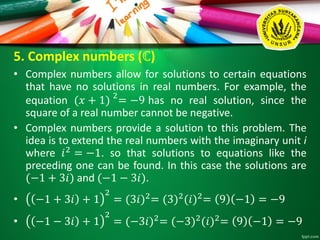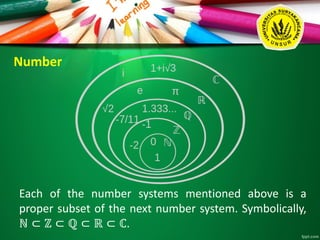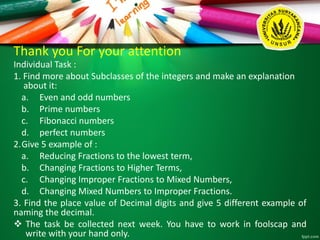1 de 21

### Secondary Lecture

• 1. English For Math Suryakancana University, Second Lecture
• 2. Numeral system • A numeral system (or system of numeration) is a writing system for expressing numbers, that is, a mathematical notation for representing numbers of a given set, using digits or other symbols in a consistent manner. Numerical digit • A digit is a numeric symbol (such as "2" or "5") used in combinations (such as "25") to represent numbers (such as the number 25) in positional numeral systems. For example, the decimal system (base 10) has ten digits (0 through to 9), whereas binary (base 2) has two digits (0 and 1).
• 3. Positional systems in detail • In a positional base-b numeral system (with b a natural number greater than 1 known as the radix or base), b basic symbols (or digits) corresponding to the first b natural numbers including zero are used. For example, for the decimal system (the most common system in use today) the radix is ten, because it uses the ten digits from 0 through 9. • the numeral (4327)10 means (4 × 103) + (3 × 102) + (2 × 101) + (7 × 100), noting that 100 = 1. • Another example is (100)10 (in the decimal system) represents the number one hundred, whilst (100)2, (in the binary system with base 2) represents the number four. 1 × 22 + 0 × 21 + 0 × 20 = 4.
• 5. Number • A number is a mathematical object used to count, measure and label. The original examples are the natural numbers 1, 2, 3, and so forth. A notational symbol that represents a number is called a numeral. In addition to their use in counting and measuring, numerals are often used for labels (as with telephone numbers), for ordering (as with serial numbers), and for codes (as with ISBNs). In common usage, the term number may refer to a symbol, a word or a mathematical abstraction.
• 6. Number Venn Diagram Subsets of the complex numbers.
• 7. Main classification Different types of numbers have many different uses. Numbers can be classified into sets, called number systems, such as the natural numbers and the real numbers.
• 8. 1. Natural numbers (ℕ) • In mathematics, the natural numbers (sometimes called the whole numbers if 0 is included) The most familiar numbers are the natural numbers or counting numbers: 1, 2, 3, and so on. Traditionally, the sequence of natural numbers started with 1. The mathematical symbol for the set of all natural numbers is ℕ. • In the base 10 numeral system, in almost universal use today for mathematical operations, the symbols for natural numbers are written using ten digits. It consists of 10 symbols or digits, 0, 1, 2, 3, 4, 5, 6, 7, 8, and 9, which are used to make our numbers. Each digit in a number has a place value.
• 9. 1. Natural numbers (ℕ) • the rightmost digit of a natural number has a place value of 1, and every other digit has a place value ten times that of the place value of the digit to its right.
• 10. 2. Integers (ℤ) • we used the set of whole numbers which consists of the numbers 0, 1, 2, 3, 4, 5, . . . . In algebra we extend the set of whole numbers by adding the negative numbers -1, -2, -3, -4, -5, . . . . The numbers . . . -5, -4, -3, -2, -1, 0 1, 2, 3, 4, 5, . . . are called integers, written as ℤ. The number zero is called the origin. • Each integer has an opposite. The opposite of a given integer is the corresponding integer, which is exactly the same distance from the origin as the given integer. For example, the opposite of -4 is +4 or 4. The opposite of 0 is 0. • The positive distance any number is from 0 is called the absolute value of the number. The symbol for absolute value is | |. Hence, | − 6| = 6 and | + 10| = 10. In other words, the absolute value of any number except 0 is positive. The absolute value of 0 is 0, i.e., |0| = 0.
• 11. 2. Integers (ℤ) • The natural numbers form a subset of the integers. the natural numbers without zero are commonly referred to as positive integers, and the natural numbers with zero are referred to as non-negative integers. • When the same number is multiplied by itself, the indicated product can be written in exponential notation. For example, 3 × 3 can be written as 32 , there the 3 is called the base and the 2 is called the exponent. • 32 is read as ‘‘three squared’’ or ‘‘three to the second power’’, 33 is read as ‘‘three cubed’’ or ‘‘three to the third power’’, 34 is read as ‘‘three to the fourth power,’’ etc.
• 12. 2. Order of Operations Integers (ℤ) In mathematics, we have what is called an order of operations to clarify the meaning when there are operations and grouping symbols (parentheses) in the same problem. The order of operations is : 1. Parentheses 2. Exponents 3. Multiplication or Division, left to right 4. Addition or Subtraction, left to right Multiplication and division are equal in order and should be performed from left to right. Addition and subtraction are equal in order and should be performed from left to right. • Math Note: The word simplify means to perform the operations following the order of operations.
• 13. 3. Rational numbers (ℚ) • A rational number is a number that can be expressed as a fraction with an integer numerator and a positive integer denominator. Fractions are written as two integers, the numerator and the denominator, with a dividing bar between them. The fraction 𝑚 𝑛 represents m parts of a whole divided into n equal parts, where n is not zero. m is also called dividend, and n is also called divisor. Two different fractions may correspond to the same rational number; for example 1 2 and 2 4 are equal. • The symbol for the rational numbers is ℚ (for quotient).
• 14. 3. Rational numbers (ℚ) • A fraction whose numerator is less than its denominator is called a proper fraction. For example, 5 8 , 2 3 , 𝑎𝑛𝑑 1 6 . A fraction whose numerator is greater than or equal to its denominator is called an improper fraction. For example, 5 3 , 6 6 , 𝑎𝑛𝑑 10 4 . A number that consists of a whole number and a fraction is called a mixed number. For example, 6 5 8 , 8 2 3 , 𝑎𝑛𝑑 3 1 6 . When the numerator of a fraction is zero, the value of the fraction is zero. For example, 0 6 = 0. • A real number that is not rational is called irrational. Irrational numbers include 2, 𝜋, ℮, and 𝜑.
• 15. 4. Real numbers (ℝ) • A real number may be either rational or irrational; either algebraic or transcendental; and either positive, negative, or zero (Integers). They may be expressed by decimal representations that have an infinite sequence of digits to the right of the decimal point, in which a decimal point is placed to the right of the digit with place value 1. Each digit to the right of the decimal point has a place value one- tenth of the place value of the digit to its left. For example, 123.456 represents 123456 1000 , or, in words, one hundred, two tens, three ones, four tenths, five hundredths, and six thousandths. • The symbol for the real numbers is ℝ.
• 16. 4. Real numbers (ℝ) • One half is 0.5, one fifth is 0.2, one tenth is 0.1, and one fiftieth is 0.02. To represent the rest of the real numbers requires an infinite sequence of digits after the decimal point. Since it impossible to write infinitely many digits, real numbers are commonly represented by rounding or truncating this sequence, or by establishing a pattern, such as 0.333..., with an ellipsis to indicate that the pattern continues. Thus 123.456 is an approximation of any real number between 123456 1000 or 123456 1000 (rounding) or any real number between 123456 1000 or 123456 1000 (truncation).
• 17. 4. Real numbers (ℝ) • The real numbers include all the rational numbers, such as the integer and the fraction, and all the irrational numbers such as 2, 𝜋, ℮, and 𝜑. • Real numbers can be thought of as points on an infinitely long line called the number line or real line, where the points corresponding to integers are equally spaced. The real line can be thought of as a part of the complex plane, and complex numbers include real numbers. Number Line : Real numbers can be thought of as points on an infinitely long number line
• 18. 5. Complex numbers (ℂ) • Moving to a greater level of abstraction, the real numbers can be extended to the complex numbers. This set of numbers arose historically from trying to find closed formulas for the roots of cubic and quartic polynomials. This led to expressions involving the square roots of negative numbers, and eventually to the definition of a new number: a square root of −1, denoted by i, a symbol assigned by Leonhard Euler, and called the imaginary unit. • The complex numbers consist of all numbers of the form 𝑎 + 𝑏𝑖, where a and b are real numbers, and i is the imaginary unit, that satisfies the equation 𝑖2 = −1.
• 19. 5. Complex numbers (ℂ) • Complex numbers allow for solutions to certain equations that have no solutions in real numbers. For example, the equation (𝑥 + 1) 2 = −9 has no real solution, since the square of a real number cannot be negative. • Complex numbers provide a solution to this problem. The idea is to extend the real numbers with the imaginary unit i where 𝑖2 = −1. so that solutions to equations like the preceding one can be found. In this case the solutions are (−1 + 3𝑖) and (−1 − 3𝑖). • −1 + 3𝑖 + 1 2 = (3𝑖)2 = (3)2 (𝑖)2 = 9 −1 = −9 • −1 − 3𝑖 + 1 2 = (−3𝑖)2 = (−3)2 (𝑖)2 = 9 −1 = −9
• 20. Number Each of the number systems mentioned above is a proper subset of the next number system. Symbolically, ℕ ⊂ ℤ ⊂ ℚ ⊂ ℝ ⊂ ℂ.
• 21. Thank you For your attention Individual Task : 1. Find more about Subclasses of the integers and make an explanation about it: a. Even and odd numbers b. Prime numbers c. Fibonacci numbers d. perfect numbers 2.Give 5 example of : a. Reducing Fractions to the lowest term, b. Changing Fractions to Higher Terms, c. Changing Improper Fractions to Mixed Numbers, d. Changing Mixed Numbers to Improper Fractions. 3. Find the place value of Decimal digits and give 5 different example of naming the decimal.  The task be collected next week. You have to work in foolscap and write with your hand only.